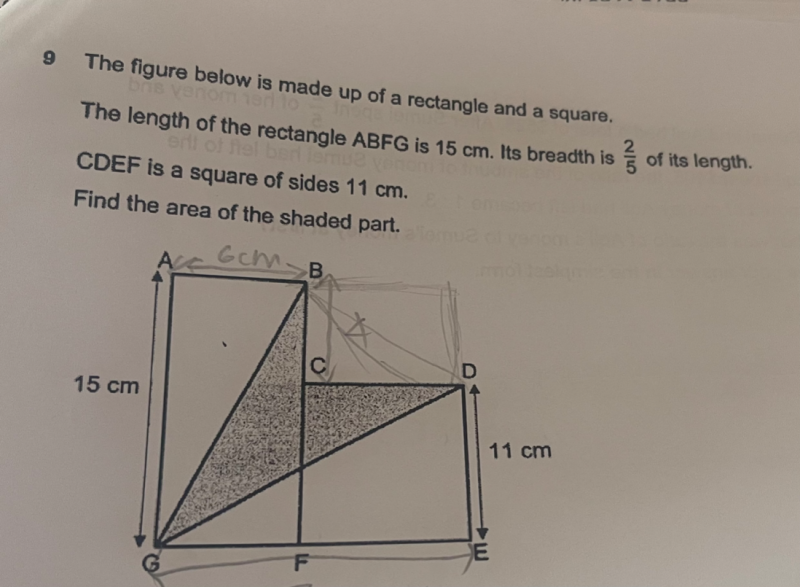# QuestionHi, please assist us on the above question. Thank you

Alternative Solution:

Area of rectangle + Area of square = (15 x 6) + (11 x 11 ) = 90 + 121 = 211

Area of triangle ABG + Area of triangle DEG = (1/2)(6)(15) + (1/2)(17)(11) = 45 + 93.5 = 138.5

Hence Area of shaded part = 211 – 138.5 = 72.5cm2

0 Replies 1 Like ✔Accepted Answer

0 Replies 0 Likes

FE = 11 cm
GE = 11 + 6 = 17 cm

Area of triangle ABG = 1/2(15)(6) = 45 cm²

Area of triangle DGE = 1/2(17)(11) = 93.5 cm²

Area of rectangle BCDO =11*4 = 44 cm²

Area of overall rectangle AOEG = 15*17 = 255 cm²

So area of shaded parts = 255 – 45 – 93.5 – 44 = 72.5 cm² #

PS.  Indeed I made an error with DGE, thanks @sushi88!  Corrected my working.

1 Reply 1 Like